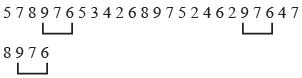Courses

# Olympiad Test: Number Ranking Test - 1

## 10 Questions MCQ Test Mathematics Olympiad Class 7 | Olympiad Test: Number Ranking Test - 1

Description
This mock test of Olympiad Test: Number Ranking Test - 1 for Class 7 helps you for every Class 7 entrance exam. This contains 10 Multiple Choice Questions for Class 7 Olympiad Test: Number Ranking Test - 1 (mcq) to study with solutions a complete question bank. The solved questions answers in this Olympiad Test: Number Ranking Test - 1 quiz give you a good mix of easy questions and tough questions. Class 7 students definitely take this Olympiad Test: Number Ranking Test - 1 exercise for a better result in the exam. You can find other Olympiad Test: Number Ranking Test - 1 extra questions, long questions & short questions for Class 7 on EduRev as well by searching above.
QUESTION: 1

### Direction: Study the given number series and answer the questions based on it. 5 7 8 9 7 6 5 3 4 2 6 8 9 7 5 2 4 6 2 9 7 6 4 7 8 9 7 6  Q. How many 7s are preceded by 9 and followed by 6?

Solution:QUESTION: 2

### Direction: Study the given number series and answer the questions based on it. 5 7 8 9 7 6 5 3 4 2 6 8 9 7 5 2 4 6 2 9 7 6 4 7 8 9 7 6  Q. Which digits have equal frequency?

Solution:

2, 5, 8 all have frequency 3.

QUESTION: 3

### Direction: Study the given number series and answer the questions based on it. 5 7 8 9 7 6 5 3 4 2 6 8 9 7 5 2 4 6 2 9 7 6 4 7 8 9 7 6  Q. Which digit has highest frequency?

Solution:

7 has highest frequency.

QUESTION: 4

Direction: Study the given number series and answer the questions based on it.
5 7 8 9 7 6 5 3 4 2 6 8 9 7 5 2 4 6 2 9 7 6 4 7 8 9 7 6
Q. Which digit has lowest frequency?

Solution:

3 has lowest frequency.

QUESTION: 5

Direction: Study the given number series and answer the questions based on it.
5 7 8 9 7 6 5 3 4 2 6 8 9 7 5 2 4 6 2 9 7 6 4 7 8 9 7 6
Q. Which digit occurs five times?

Solution:

6 occurs five times.

QUESTION: 6

Direction: Study the given number series and answer the questions based on it.
5 7 8 9 7 6 5 3 4 2 6 8 9 7 5 2 4 6 2 9 7 6 4 7 8 9 7 6
Q. How many times an even number is preceded by an odd number?

Solution:
QUESTION: 7

Direction: Study the given number series and answer the questions based on it.
5 7 8 9 7 6 5 3 4 2 6 8 9 7 5 2 4 6 2 9 7 6 4 7 8 9 7 6
Q. How many times two consecutive digits are odd numbers?

Solution:
QUESTION: 8

Direction: Study the given number series and answer the questions based on it.
5 7 8 9 7 6 5 3 4 2 6 8 9 7 5 2 4 6 2 9 7 6 4 7 8 9 7 6
Q. How many times digit 9 occurs?

Solution:
QUESTION: 9

Direction: Study the following five numbers and answer the questions given below:
617, 325, 639, 841, 592
Q. What will be the first digit of the second highest number after the positions of only the second and third digits within each number are interchanged?

Solution:

671, 352, 693, 814, 529
The numbers in the descending order, 814, 693, 671, 529, 352
Second highest number = 693
Its 1st digit = 6

QUESTION: 10

Direction: Study the following five numbers and answer the questions given below :
617, 325, 639, 841, 592
Q. What will be the last digit of the third number from bottom when they are arranged in descending order after reversing the position of the digits within each number?

Solution:

716, 523, 936, 148, 295
The numbers in the descending order 936, 716, 523, 295, 148.
Third number from bottom = 52 3